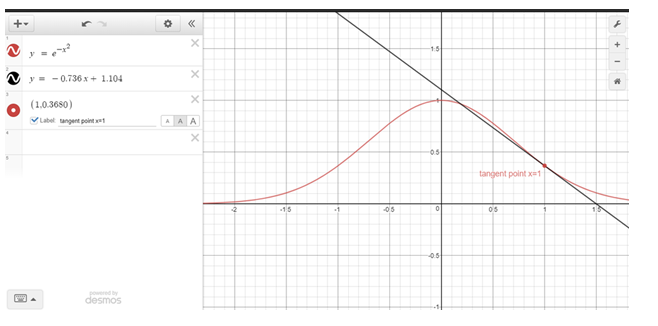# To estimate the value of f ' ( 1 ) for the given function graphically and numerically.### Single Variable Calculus: Concepts...

4th Edition
James Stewart
Publisher: Cengage Learning
ISBN: 9781337687805### Single Variable Calculus: Concepts...

4th Edition
James Stewart
Publisher: Cengage Learning
ISBN: 9781337687805

#### Solutions

Chapter 2, Problem 31RE

a.

To determine

## To estimate the value of f'(1) for the given function graphically and numerically.

Expert Solution

0.7357

### Explanation of Solution

Given:

The given function is f(x)=ex2

Calculation:

The following is the graph of the given function-The equation of f'(x) is 2xex2 value which would be approx 0.7357 .

b.

To determine

### To find an approximation equation of the tangent line to the curve y=e−x2 at the point where x=1 .

Expert Solution

y=0.36780.7357(x1)

### Explanation of Solution

Given:

The given function is f(x)=ex2

Calculation:

The value of f'(1)=0.73575 and the value of f(1)=0.3678

So, tangent line y=0.36780.7357(x1)

c.

To determine

Expert Solution

### Explanation of Solution

Given:

The given function is f(x)=ex2

Calculation:

The following is the graph of the given function-### Have a homework question?

Subscribe to bartleby learn! Ask subject matter experts 30 homework questions each month. Plus, you’ll have access to millions of step-by-step textbook answers!